Projection onto Non-Orthogonal Vectors Next  |  Prev  |  Up  |  Top  |  Index  |  JOS Index  |  JOS Pubs  |  JOS Home  |  Search

### Projection onto Non-Orthogonal Vectors

Consider this example: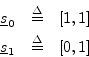These point in different directions, but they are not orthogonal. What happens now? The projections are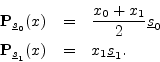The sum of the projections is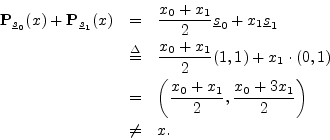So, even though the vectors are linearly independent, the sum of projections onto them does not reconstruct the original vector. Since the sum of projections worked in the orthogonal case, and since orthogonality implies linear independence, we might conjecture at this point that the sum of projections onto a set of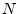vectors will reconstruct the original vector only when the vector set is orthogonal, and this is true, as we will show.

It turns out that one can apply an orthogonalizing process, called Gram-Schmidt orthogonalization to anylinearly independent vectors in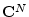so as to form an orthogonal set which will always work. This will be derived in Section 5.10.4.

Obviously, there must be at leastvectors in the set. Otherwise, there would be too few degrees of freedom to represent an arbitrary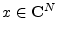. That is, given thecoordinates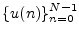of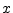(which are scale factors relative to the coordinate vectors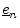in), we have to find at leastcoefficients of projection (which we may think of as coordinates relative to new coordinate vectors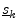). If we compute only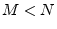coefficients, then we would be mapping a set ofcomplex numbers tonumbers. Such a mapping cannot be invertible in general. It also turns outlinearly independent vectors is always sufficient. The next section will summarize the general results along these lines.

Next  |  Prev  |  Up  |  Top  |  Index  |  JOS Index  |  JOS Pubs  |  JOS Home  |  Search

[How to cite this work] [Order a printed hardcopy]